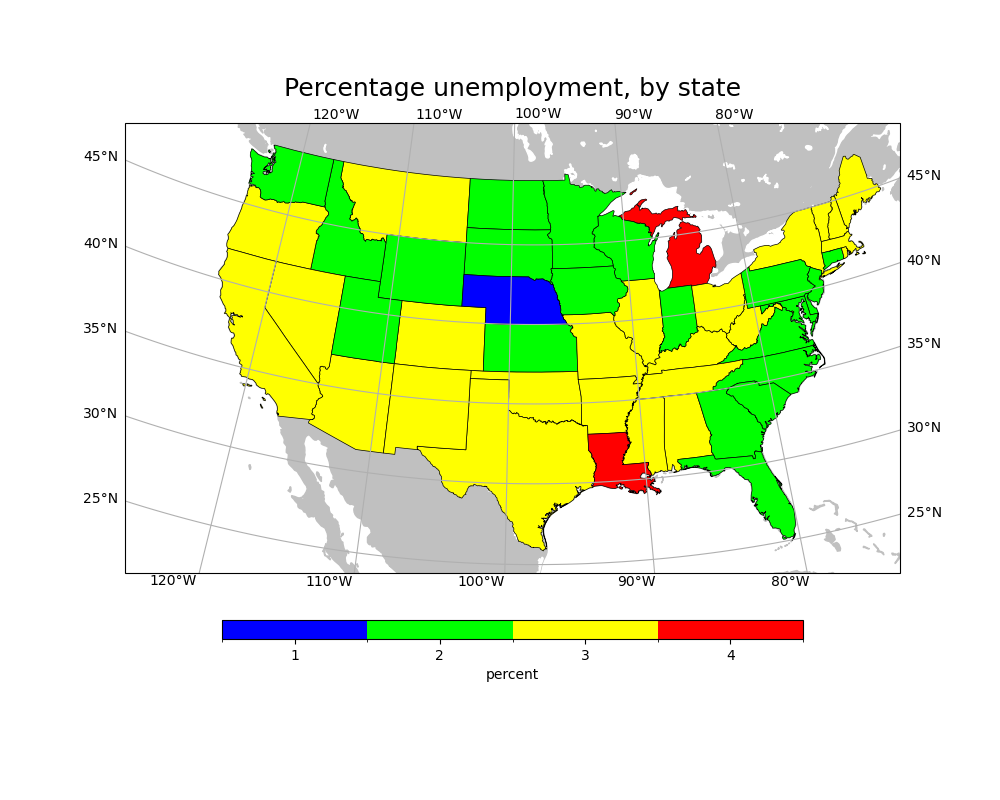# NCL_shapefiles_1.py#

This script illustrates the following concepts:

• Plotting data from shapefiles

• Using shapefile data to plot unemployment percentages in the U.S.

• Drawing a custom colorbar on a map

• Drawing filled polygons over a Lambert Conformal plot

• Drawing the US with a Lambert Conformal projection

• Zooming in on a particular area on a Lambert Conformal map

• Centering the labels under the colorbar boxes

See following URLs to see the reproduced NCL plot & script:
Note:

At the time of making this example, there isn’t a good way to draw tick marks along with the latitude and longitude labels. We have chosen to draw gridlines to show exactly where the labels are pointing. The gridlines can be removed by calling `gl.xlines = False` and `gl.ylines = False` after drawing the labels.

Import packages:

```import matplotlib.pyplot as plt
import matplotlib.patches as mpatches
import matplotlib.colors as colors
import matplotlib.cm as cm
import matplotlib.ticker as mticker
import shapefile as shp
import numpy as np
import cartopy.crs as ccrs
import cartopy.feature as cfeature

import geocat.datafiles as gdf
import geocat.viz as gv
```

```# Open all shapefiles and associated .dbf, .shp, and .prj files
open(gdf.get("shape_files/states.dbf"), 'r')
open(gdf.get("shape_files/states.shp"), 'r')
open(gdf.get("shape_files/states.shx"), 'r')
open(gdf.get("shape_files/states.prj"), 'r')

# Open shapefiles
```

Set color map colors and bounds

```colormap = colors.ListedColormap(['blue', 'lime', 'yellow', 'red'])

colorbounds = [0.5, 1.5, 2.5, 3.5, 4.5]

norm = colors.BoundaryNorm(colorbounds, colormap.N)
```

Helper function to determine state color:

```def color_assignment(record):
population = record.PERSONS
unempolyment = record.UNEMPLOY
percent = unempolyment / population
if (0.01 <= percent and percent < 0.02):
return colormap.colors
elif (0.02 <= percent and percent < 0.03):
return colormap.colors
elif (0.03 <= percent and percent < 0.04):
return colormap.colors
elif (0.04 <= percent):
return colormap.colors
```

Plot:

```plt.figure(figsize=(10, 8))
ax = plt.axes(projection=ccrs.LambertConformal(standard_parallels=(33, 45),
central_longitude=-98))
ax.set_extent([-125, -74, 22, 50])

for i in range(0, len(shapefile.shapes())):
shape = shapefile.shape(i)
record = shapefile.record(i)
color = color_assignment(record)
# if a shape has multiple parts make each one a separate patch
if len(shape.parts) > 1:
for j in range(0, len(shape.parts)):
start_index = shape.parts[j]
# the last part uses the remaining points and doesn't require and end_index
if (j is (len(shape.parts) - 1)):
patch = mpatches.Polygon(shape.points[start_index:],
facecolor=color,
edgecolor='black',
linewidth=0.5,
transform=ccrs.PlateCarree(),
zorder=2)
else:
end_index = shape.parts[j + 1]
patch = mpatches.Polygon(shape.points[start_index:end_index],
facecolor=color,
edgecolor='black',
linewidth=0.5,
transform=ccrs.PlateCarree(),
zorder=2)
else:
patch = mpatches.Polygon(shape.points,
facecolor=color,
edgecolor='black',
linewidth=0.5,
transform=ccrs.PlateCarree(),
zorder=2)

# Create colorbar
plt.colorbar(cm.ScalarMappable(cmap=colormap, norm=norm),
ax=ax,
boundaries=colorbounds,
orientation='horizontal',
shrink=0.75,
ticks=[1, 2, 3, 4],
label='percent',
aspect=30,

# Add latitude and longitude labels
gl = ax.gridlines(draw_labels=True, x_inline=False, y_inline=False)
gl.xlocator = mticker.FixedLocator(np.linspace(-120, -80, 5))
gl.ylocator = mticker.FixedLocator(np.linspace(25, 45, 5))
gl.xlabel_style = {'rotation': 0}
gl.ylabel_style = {'rotation': 0}

# Use geocat.viz.util convenience function to set titles and labels
gv.set_titles_and_labels(ax, maintitle='Percentage unemployment, by state')

plt.show()
```Total running time of the script: ( 0 minutes 2.583 seconds)

Gallery generated by Sphinx-Gallery